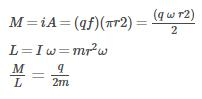Courses

# Test: Magnetic Properties

## 10 Questions MCQ Test Physics Class 12 | Test: Magnetic Properties

Description
This mock test of Test: Magnetic Properties for JEE helps you for every JEE entrance exam. This contains 10 Multiple Choice Questions for JEE Test: Magnetic Properties (mcq) to study with solutions a complete question bank. The solved questions answers in this Test: Magnetic Properties quiz give you a good mix of easy questions and tough questions. JEE students definitely take this Test: Magnetic Properties exercise for a better result in the exam. You can find other Test: Magnetic Properties extra questions, long questions & short questions for JEE on EduRev as well by searching above.
QUESTION: 1

### What is the unit of susceptibility?​

Solution:

In electromagnetism the magnetic susceptibility (Latin: susceptibilis, “receptive”; denoted X) is a measure of how much a material will become magnetized in an applied magnetic field. Mathematically, it is the ratio of magnetization M(magnetic moment per unit volume) to  the applied magnetizing field intensity H.

QUESTION: 2

### The magnetic induction left behind in the sample after the magnetizing field has been removed is called

Solution:

Remanence or remanent magnetization or residual magnetism is the magnetization left behind in a ferromagnetic material (such as iron) after an external magnetic field is removed. It is also the measure of that magnetization. Colloquially, when a magnet is "magnetized" it has remanence.The remanence of magnetic materials provides the magnetic memory in magnetic storage devices, and is used as a source of information on the past Earth's magnetic field in paleomagnetism.The equivalent term residual magnetization is generally used in engineering applications. In transformers, electric motors and generators a large residual magnetization is not desirable (see also electrical steel) as it is an unwanted contamination, for example a magnetization remaining in an electromagnet after the current in the coil is turned off. Where it is unwanted, it can be removed by degaussing.Sometimes the term retentivity is used for remanence measured in units of magnetic flux density.

QUESTION: 3

### Declination is the angle between:

Solution:

Magnetic declination is the angle on the horizontal plane between magnetic north (the direction the north end of a compass needle points, corresponding to the direction of the Earth's magnetic field lines) and true north (the direction along a meridian towards the geographic North Pole).

QUESTION: 4

The ferromagnetic materials can be magnetised easily because

Solution:

Such materials are called ferromagnetic, after the Latin word for iron, ferrum. Not only do ferromagnetic materials respond strongly to magnets (the way iron is attracted to magnets), they can also be magnetized themselves—that is, they can be induced to be magnetic or made into permanent magnets.

QUESTION: 5

What kinds of materials are used for coating magnetic tapes?

Solution:

Ceramics are used for coating magnetic tapes in a cassette player or for building memory stores in a modern computer. Ceramics are specially treated barium-iron oxides and are also called ferrites.

QUESTION: 6

A particle of charge q and mass m moves in a circular orbit of radius r with angular speed. The ratio of the magnitude of its magnetic moment to that of its angular momentum depends on

Solution:

To find the magnetic moment due to a single particle, it is advisable to consider the equivalent current due to itQUESTION: 7

A wire of length L m carrying current i A is bent in the form of a circle. The magnitude of magnetic moment is

Solution:

If r is the radius of the circle,
then L=2πr or, r=L/2π
Area=A=πr2=πL2/4π2​= L2​/4π
Magnetic Moment=IA= IL2/4π

QUESTION: 8

If the susceptibility of a metal at saturation is 4500, then what is the permeability of this metal at saturation?​

Solution:

μr=μ/μ0
μ= μr x μ0
μ=4500 x 4πx 10-7
μ=56520x10-7
μ=5.652x10-3

QUESTION: 9

The maximum value of permeability of a metal (77% Ni , 16% Fe , 5% Cu , 2% Cr) is 0.164 T-m/A. What will be the maximum relative permeability and susceptibility?​

Solution:
QUESTION: 10

The units of magnetic pole strength and magnetic dipole moment of a bar magnet are

Solution:

The units of magnetic pole strength is (A-m) and magnetic dipole moment of a bar magnet is (JT-1)